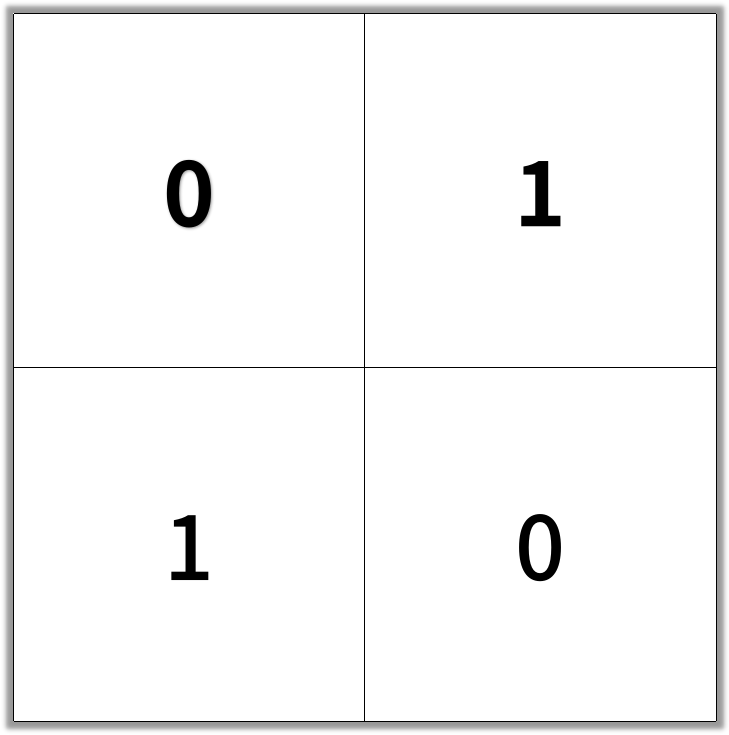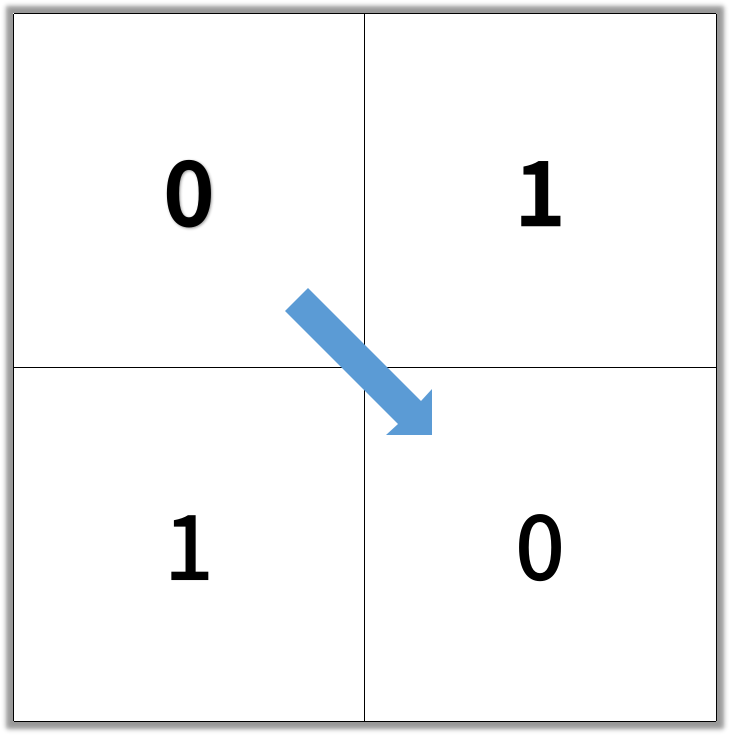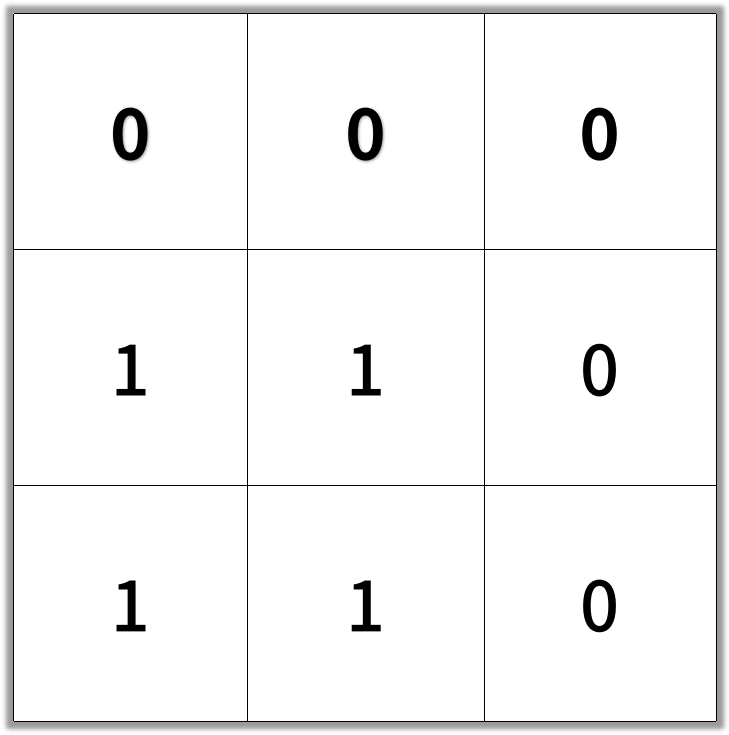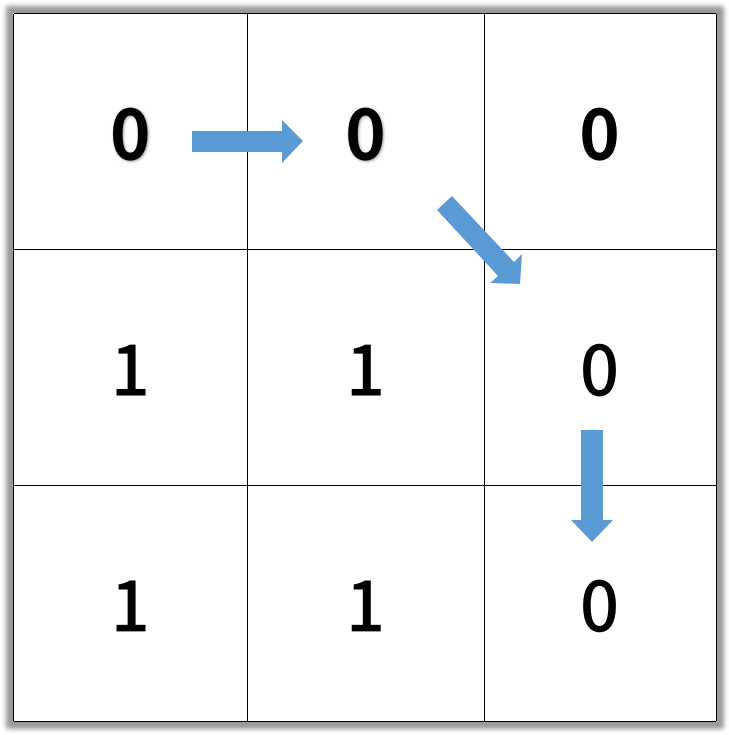# 二进制矩阵中的最短路径

## 1091. 二进制矩阵中的最短路径 (Medium)

• 相邻单元格 `C_i` 和 `C_{i+1}` 在八个方向之一上连通（此时，`C_i` 和 `C_{i+1}` 不同且共享边或角）
• `C_1` 位于 `(0, 0)`（即，值为 `grid`
• `C_k` 位于 `(N-1, N-1)`（即，值为 `grid[N-1][N-1]`
• 如果 `C_i` 位于 `(r, c)`，则 `grid[r][c]` 为空（即，`grid[r][c] == 0`

```输入：[[0,1],[1,0]]```

```输入：[[0,0,0],[1,1,0],[1,1,0]]```

1. `1 <= grid.length == grid.length <= 100`
2. `grid[i][j]` 为 `0` 或 `1`

[广度优先搜索]